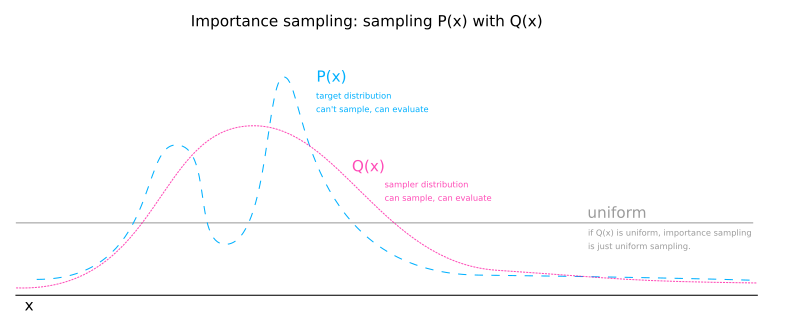Math and science::INF ML AI

# Importance sampling

Let $$P(x)$$ be a distribution which we can evaluate an unnormalized version easily at a point to obtain $$P^*(x)$$. $$P(x) = \frac{P^*(x)}{Z}$$ where $$Z = \int P^*(x) \dd{x}$$. Now propose we have a function $$\phi(x)$$ for which we wish to evaluate an expectation. We may proceed with uniform sampling by taking $$R$$ samples of a uniform distribution over the domain, $$x_1, x_2, ... x_R$$ and calculating an estimate:

[$\mathrm{E}[\phi] = \frac{?}{?}$]

Importance sampling alters this calculation by sampling domain values from a distribution other than the uniform distribution. Let $$Q(x)$$ be the sampler distribution. We will sample $$R$$ samples from the sampler distribution and estimate the expectation of $$\phi$$ like so:

todo

$\mathrm{E}[\phi] = \frac{}$The sampler distribution has two effects:

1. It focuses where in the domain $$x$$ is sampled.
2. It causes the estimation of the normalizing $$Z_P$$ constant to be skewed by oversampling some values and undersampling others.

The first effect is beneficial and the second is not. The second effect can be conceptualised  as $$Q(x)$$ stretching the domain in some places and shirking it in others. The size of the domain is important as a measure by which we are weighting the $$P^*(x)$$ that we are collecting. To counteract the undesired effect of $$Q(x)$$, we simply multiply $$P^*(x)$$ by $$\frac{1}{Q(x)$$. Any part of the domain that is stretch by $$Q(x)$$ shrinks the weight given to a $$P^*(x)$$ sample by multiplying by the reciprocal amount.

The fact that we multiply $$\phi(x)$$ by $$P^*(x)$$ fully accounts for the weighting for $$\phi$$. The $$\frac{1}{Q(x_0)$$ factor is simply to undo the fact that we are going to be sampling point $$x_0$$ excessively according to the value $$Q(x_0)$$.